TitleIntermediate Algebra Tutorial 2: Algebraic Expressions

WTAMU > Virtual Math Lab > Intermediate AlgebraLearning Objectives

 After completing this tutorial, you should be able to: Know what a variable is. Know what an algebraic expression is. Evaluate an algebraic expression given a value for a variable. Translate an English phrase into an algebraic expressionIntroduction

 This tutorial will go over some key definitions and phrases used when specifically working with algebraic expressions as well as evaluating them.    It is very IMPORTANT that you understand some of the math lingo that is used in an algebra class, otherwise it may all seem Greek to you. Knowing the terms and concepts on this page will definitely help you build an understanding of  what a variable is and get you more comfortable working with them.  Variables are a HUGE part of algebra, so it is very important for you to feel at ease around them in order to be successful in algebra.  So let's get going and help you get on the road to being variable savvy.Tutorial

 Variable

 A variable is a letter that represents a number. Don't let the fact that it is a letter throw you. Since it represents a number, you treat it just like you do a number when you do various mathematical operations involving variables. x is a very common variable that is used in algebra, but you can use any letter (a, b, c, d, ....) to be a variable.

 Algebraic Expressions

 An algebraic expression is a number, variable or combination of the two connected by some mathematical operation like addition, subtraction, multiplication, division, exponents, and/or roots. 2x + y, a/5, and 10 - r are all examples of algebraic expressions.

 Evaluating an Expression

 You evaluate an expression by replacing the variable with the given number and performing the indicated operation.

 Value of an Expression

 When you are asked to find the value of an expression, that means you are looking for the result that you get when you evaluate the expression.

 So keep in mind that vary means to change - a variable allows an expression to take on different values, depending on the situation.  For example, the area of a rectangle is length times width.  Well, not every rectangle is going to have the same length and width, so we can use an algebraic expression with variables to represent the area and then plug in the appropriate numbers to evaluate it.  So if we let the length be the variable l and width be w, we can use the expression lw.  If a given rectangle has a length of 4 and width of 3, we would evaluate the expression by replacing l with 4 and w with 3 and multiplying to get a value of 4 times 3 or 12. Let's step through some examples that help illustrate these ideas.Example 1:  Find the value of the algebraic expression at the given value.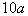when a = 15.

 Remember that when a number is directly in front of a variable (there is no + or - between them), like in this example, that means that when you evaluate this expression you will be multiplying 10 times whatever value a is. Replace a with 15 and then multiply: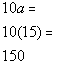*Replace a with 15 *(  ) is another way to write mult. *Multiply together

 That wasn't so bad, let's look at another example.Example 2:   Find the value of the algebraic expression at the given value.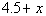when x = 3.2

 Replace x with 3.2 and then add:*Replace x with 3.2 *Add the numbers

 Sometimes, there are more than one variable involved, you just extend this basic idea to how ever many variable you have.  For each variable, you simply replace it with it's corresponding value, as this next example illustrates.Example 3:  Find the value of the algebraic expression at the given value.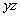whenand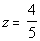Keep in mind that when you see two variables written side by side, like y and z are in this example, that means that y and z are being multiplied together. Replace y with 1/3 and z with 4/5 and multiply: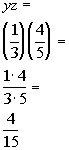*Replace y with 1/3 and z with 4/5       *Multiply the fractions together

 Note that if you need to review multiplying fractions go to this link: fractionsExample 4:   If it costs \$15 per cd at a local Entertainment store, then we can use the algebraic expression 15x, where x represents the number of cd's purchased, to find the cost of buying x cd's.  How much would it cost to buy 7?

 Even though the information was given in a little bit different form than the first three examples, it still uses the same basic concept - replace the variable with the given value and then evaluate the expression.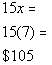*Replace x with 7 *Multiply together

 Translating an  English Phrase Into an  Algebraic Expression

 Sometimes, you find yourself having to write out your own algebraic expression based on the wording of a problem.  In that situation, you want to  read the problem carefully, pick out key words and phrases and determine their equivalent mathematical meaning, replace any unknowns with a variable, and put it all together in an algebraic expression. The following are some key words and phrases and their translations:

 Addition: sum, plus, add to, more than, increased by, total

 Subtraction:  difference of, minus, subtracted from, less than, decreased by, less

 Multiplication:  product, times, multiply, twice, of

 Division:  quotient divide, into, ratioExample 5:    Write the phrase as an algebraic expression.   The sum of a number and 10.

 In this example, we are not evaluating an expression, so we will not be coming up with a value like we did in examples 1 - 4 above.  However, we are wanting to rewrite it as an algebraic expression. It looks like the only reference to a mathematical operation is the word sum - so what operation will we have in this expression?   If you said addition, you are correct!!! The phrase 'a number' indicates that it is an unknown number - there was no specific value given to it.   So we will replace the phrase 'a number' with the variable x.  We want to let our variable represent any number that is unknown Putting everything together, we can translate the given english phrase with the following algebraic expression:

 The sum of a number and 10*'sum' = + *'a number' = variable x

 Let's try another!Example 6:  Write the phrase as an algebraic expression. The product of 5 and a number.

 Again, we are wanting to rewrite this as an algebraic expression, not evaluate it. This time, the phrase that correlates with our operation is 'product' - so what operation will we be doing this time?   If you said multiplication, you are right on. Again, we have the phrase 'a number', which again is going to be replaced with a variable, since we do not know what the number is.   Let's see what we get for this answer:

 The product of 5 and a number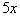*'product' = multiplication *'a number' = variable xExample 7:  Write the phrase as an algebraic expression.  3 less than twice a number

 First of all, we have the phrase 'less than' which mathematically translates as subtraction.  You need to be careful with this phrase,  it is very tempting to start off with 3 and put your subtraction sign after the 3.  However, think about it, if you want 3 less than something, you are 3 below it. In order to be 3 below something, you would have to subtract the 3!!!  So you would not have 3 minus, but minus 3 as PART of your expression. The other part of the expression involves the phrase 'twice a number'.  'Twice' translates as two times a number and, as above, we will replace the phrase 'a number' with our variable x.   Putting this together we get:

 3 less than twice a number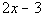*'less than' = - *'twice' = 2 times *'a number' = variable xExample 8:  Write the phrase as an algebraic expression.  The quotient of 3 and the difference of a number and 2.

 First of all, the term 'quotient' is going to be replaced with what mathematical operation?  If you said division, you are right on the mark!! Note how 3 immediately follows the phrase 'the quotient of', this means that 3 is going to be in the numerator.  The phrase that immediately follows the word 'quotient' is going to be in the numerator of it. After the word 'and', you have the phrase 'the difference of a number and 2'.  That is the second part of your quotient which means it will go in the denominator.  And what operation will we have when we do write that difference down below?  I hope you said subtraction.   Let's see what we get when we put all of this together:

 The quotient of 3 and the difference of a number and 2.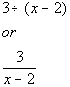*'quotient' = division *'difference' = - *'a number' = variable x

 Now it is your turn,  the next section are problems that you can practice on to see if you are understanding these topics.Practice Problems

 These are practice problems to help bring you to the next level.  It will allow you to check and see if you have an understanding of these types of problems. Math works just like anything else, if you want to get good at it, then you need to practice it.  Even the best athletes and musicians had help along the way and lots of practice, practice, practice, to get good at their sport or instrument.  In fact there is no such thing as too much practice. To get the most out of these, you should work the problem out on your own and then check your answer by clicking on the link for the answer/discussion for that  problem.  At the link you will find the answer as well as any steps that went into finding that answer.Practice Problems 1a - 1c: Find the value of the algebraic expression at the given replacement values.

 1a.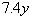when y = 2.2 (answer/discussion to 1a) 1b.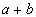when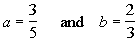(answer/discussion to 1b)

 1c.  You need to know how much area of carpet to purchase to carpet your rectangular floor.  You will need to use the algebraic expression lw to find it's area, where l represents the length of the rectangular floor and w is the width of it.  If your rectangular floor is 15 feet long and 8 feet wide, find how much area of carpet you would need to cover it. (answer/discussion to 1c)Practice Problems 2a - 2c: Write each phrase as an algebraic expression.

 2a.   15 more than a number. (answer/discussion to 2a)

 2b.   The quotient of 7 and the product of 3 and a number. (answer/discussion to 2b)

 2c.   17 less than the sum of a number and 8. (answer/discussion to 2c)Need Extra Help on these Topics?

The following are webpages that can assist you in the topics that were covered on this page:

 http://www.purplemath.com/modules/variable.htm This webpage goes over what a variable is and the uses for it. http://www.mathleague.com/help/algebra/algebra.htm#variables This webpage goes over what a variable is and the uses for it http://www.mathleague.com/help/algebra/algebra.htm#expressions This webpage will help you better understand expressions

Go to Get Help Outside the Classroom found in Tutorial 1: How to Succeed in a Math Class for some more suggestions.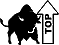Last revised on June 10, 2011 by Kim Seward.
All contents copyright (C) 2002 - 2011, WTAMU and Kim Seward. All rights reserved.